/scikit-learn

# sklearn.model_selection.KFold

`class sklearn.model_selection.KFold(n_splits=’warn’, shuffle=False, random_state=None)` [source]

K-Folds cross-validator

Provides train/test indices to split data in train/test sets. Split dataset into k consecutive folds (without shuffling by default).

Each fold is then used once as a validation while the k - 1 remaining folds form the training set.

Read more in the User Guide.

Parameters: `n_splits : int, default=3` Number of folds. Must be at least 2. Changed in version 0.20: `n_splits` default value will change from 3 to 5 in v0.22. `shuffle : boolean, optional` Whether to shuffle the data before splitting into batches. `random_state : int, RandomState instance or None, optional, default=None` If int, random_state is the seed used by the random number generator; If RandomState instance, random_state is the random number generator; If None, the random number generator is the RandomState instance used by `np.random`. Used when `shuffle` == True.

`StratifiedKFold`
Takes group information into account to avoid building folds with imbalanced class distributions (for binary or multiclass classification tasks).
`GroupKFold`
K-fold iterator variant with non-overlapping groups.
`RepeatedKFold`
Repeats K-Fold n times.

#### Notes

The first `n_samples % n_splits` folds have size `n_samples // n_splits + 1`, other folds have size `n_samples // n_splits`, where `n_samples` is the number of samples.

Randomized CV splitters may return different results for each call of split. You can make the results identical by setting `random_state` to an integer.

#### Examples

```>>> from sklearn.model_selection import KFold
>>> X = np.array([[1, 2], [3, 4], [1, 2], [3, 4]])
>>> y = np.array([1, 2, 3, 4])
>>> kf = KFold(n_splits=2)
>>> kf.get_n_splits(X)
2
>>> print(kf)
KFold(n_splits=2, random_state=None, shuffle=False)
>>> for train_index, test_index in kf.split(X):
...    print("TRAIN:", train_index, "TEST:", test_index)
...    X_train, X_test = X[train_index], X[test_index]
...    y_train, y_test = y[train_index], y[test_index]
TRAIN: [2 3] TEST: [0 1]
TRAIN: [0 1] TEST: [2 3]
```

#### Methods

 `get_n_splits`([X, y, groups]) Returns the number of splitting iterations in the cross-validator `split`(X[, y, groups]) Generate indices to split data into training and test set.
`__init__(n_splits=’warn’, shuffle=False, random_state=None)` [source]
`get_n_splits(X=None, y=None, groups=None)` [source]

Returns the number of splitting iterations in the cross-validator

Parameters: `X : object` Always ignored, exists for compatibility. `y : object` Always ignored, exists for compatibility. `groups : object` Always ignored, exists for compatibility. `n_splits : int` Returns the number of splitting iterations in the cross-validator.
`split(X, y=None, groups=None)` [source]

Generate indices to split data into training and test set.

Parameters: `X : array-like, shape (n_samples, n_features)` Training data, where n_samples is the number of samples and n_features is the number of features. `y : array-like, shape (n_samples,)` The target variable for supervised learning problems. `groups : array-like, with shape (n_samples,), optional` Group labels for the samples used while splitting the dataset into train/test set. `train : ndarray` The training set indices for that split. `test : ndarray` The testing set indices for that split.

## Examples using `sklearn.model_selection.KFold`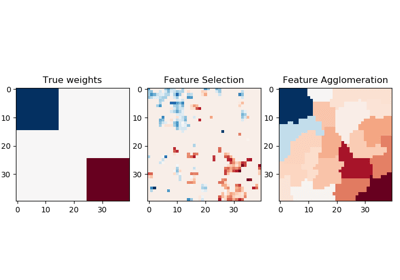Feature agglomeration vs. univariate selection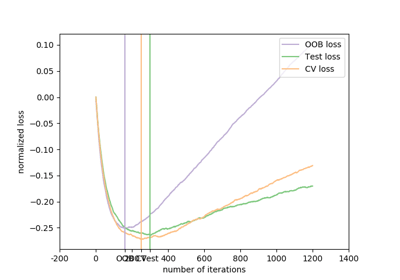Gradient Boosting Out-of-Bag estimates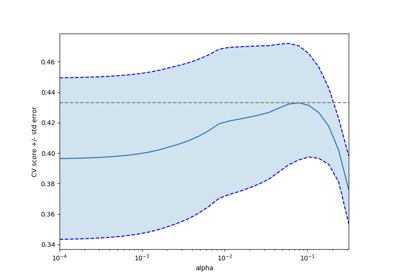Cross-validation on diabetes Dataset Exercise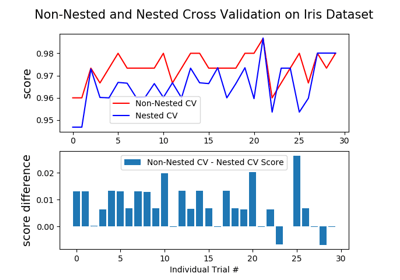Nested versus non-nested cross-validation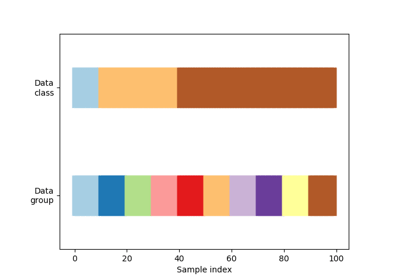Visualizing cross-validation behavior in scikit-learn

© 2007–2018 The scikit-learn developers